## r(n)

The number of representations ofbysquares is denoted. The Mathematica(Wolfram Research, Champaign, IL) function NumberTheoryNumberTheoryFunctionsSumOfSquaresR[k,n] gives.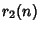is often simply written. Jacobisolved the problem for, 4, 6, and 8. The first cases, 4, and 6 were found by equating Coefficients of the Theta Function,, and. The solutions forand 12 were found by Liouville and Eisenstein, and Glaisher (1907) gives a table offor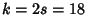.was found as a finite sum involving quadratic reciprocity symbols by Dirichlet.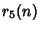and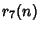were found by Eisenstein, Smith, and Minkowski.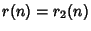is 0 wheneverhas a Prime divisor of the formto an Odd Power; it doubles upon reaching a new Prime of the form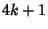. It is given explicitly by(1)

where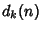is the number of Divisors ofof the form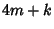. The first few values are 4, 4, 0, 4, 8, 0, 0, 4, 4, 8, 0, 0, 8, 0, 0, 4, 8, 4, 0, 8, 0, 0, 0, 0, 12, 8, 0, 0, ... (Sloane's A004018). The first few values of the summatory function(2)

are 0, 4, 8, 8, 12, 20, 20, 20, 24, 28, 36, ... (Sloane's A014198). Shanks (1993) defines instead, with. A Lambert Series foris(3)

(Hardy and Wright 1979).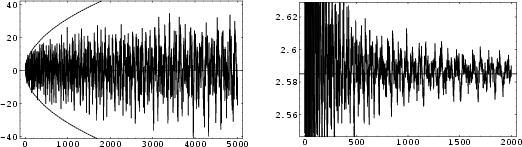Asymptotic results include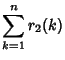(4)(5)

whereis a constant known as the Sierpinski Constant. The left plot above shows(6)

with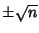illustrated by the dashed curve, and the right plot shows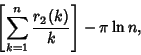(7)

with the value ofindicated as the solid horizontal line.

The number of solutions of(8)

for a givenwithout restriction on the signs or relative sizes of,, and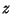is given by. Ifis Squarefree, then Gaußproved that(9)

(Arno 1992), whereis the Class Number of.

Additional higher-order identities are given by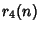(10)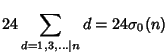(11)(12)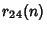(13)

where(14)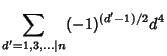(15)(16),is the number of divisors ofof the form,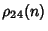is a Singular Series,is the Divisor Function,is the Divisor Function of order 0 (i.e., the number of Divisors), andis the Tau Function.

Similar expressions exist for larger Even, but they quickly become extremely complicated and can be written simply only in terms of expansions of modular functions.

See also Class Number, Landau-Ramanujan Constant, Prime Factors, Sierpinski Constant, Tau Function

References

Arno, S. The Imaginary Quadratic Fields of Class Number 4.'' Acta Arith. 60, 321-334, 1992.

Boulyguine. Comptes Rendus Paris 161, 28-30, 1915.

New York: Chelsea, p. 317, 1952.

Glaisher, J. W. L. On the Numbers of a Representation of a Number as a Sum of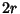Squares, whereDoes Not Exceed 18.'' Proc. London Math. Soc. 5, 479-490, 1907.

Grosswald, E. Representations of Integers as Sums of Squares. New York: Springer-Verlag, 1985.

Hardy, G. H. The Representation of Numbers as Sums of Squares.'' Ch. 9 in Ramanujan: Twelve Lectures on Subjects Suggested by His Life and Work, 3rd ed. New York: Chelsea, 1959.

Hardy, G. H. and Wright, E. M. The Function,'' Proof of the Formula for,'' The Generating Function of,'' and The Order of.'' §16.9, 16.10, 17.9, and 18.7 in An Introduction to the Theory of Numbers, 5th ed. Oxford, England: Clarendon Press, pp. 241-243, 256-258, and 270-271, 1979.

Shanks, D. Solved and Unsolved Problems in Number Theory, 4th ed. New York: Chelsea, pp. 162-153, 1993.

Sloane, N. J. A. Sequences A014198 and A004018/M3218 in An On-Line Version of the Encyclopedia of Integer Sequences.'' http://www.research.att.com/~njas/sequences/eisonline.html and Sloane, N. J. A. and Plouffe, S. The Encyclopedia of Integer Sequences. San Diego: Academic Press, 1995.